## ML Aggarwal Class 10 Solutions for ICSE Maths Chapter 1 Value Added Tax Ex 1

These Solutions are part of ML Aggarwal Class 10 Solutions for ICSE Maths. Here we have given ML Aggarwal Class 10 Solutions for ICSE Maths Chapter 1 Value Added Tax Ex 1

More Exercises

Question 1.
A manufacturing company sells a T.V. to a trader A for ₹ 18000. Trader A sells it to a trader B at a point of ₹ 750 and trader B sells it to a consumer at a profit of ₹ 900. If the rate of sales tax (under VAT) is 10%, find
(i) the amount of tax received by the Government.
(ii) the amount paid by the consumer for the T.V.
Solution:
Sale price of a T.V. to trader A = ₹ 18000
Rate of VAT tax = 10%(i) Total tax paid to Govt. = ₹ (I800 + 75 + 90) = ₹ 1965
(ii) Amount paid by the consumer to trader B = ₹ 18000 + 750 + 900 + Tax 1965 = ₹ 21615

Question 2.
A manufacturer sells a washing machine to a wholesaler for ₹ 15000. The wholesaler sells it to a trader at a profit of ₹ 1200 and the trader sells it to a consumer at a profit of ₹ 1800. If the rate of VAT is 8%, find :
(i) The amount of VAT received by the State Government on the sale of this machine from the manufacturer and the wholesaler.
(ii) The amount that the consumer pays for the machine.
Solution:
Total amount under VAT = ₹ 15000 + ₹ 1200 + ₹ 1800 = ₹ 18000
(i) VAT = 8% of ₹ 18000
= $$\frac { 8 }{ 100 }$$ x 18000 = ₹ 1440
(ii) Consumer pays for the machine = ₹ 18000 + ₹ 1440 = ₹ 19440

Question 3.
A manufacturer buys raw material for ₹ 40000 and pays sales tax at the rate of 4%. He sells the ready stock for ₹ 78000 and charges sales tax at the rate of 7.5%. Find the VAT paid by the manufacturer.
Solution:
Cost price of raw material = ₹ 40000
Rate of sales tax = 4%
Total tax = ₹ $$\frac { 40000 x 4 }{ 100 }$$ = ₹ 1600
Selling price = ₹ 78000
Rate of sales tax = 7.5%VAT paid by the manufacturer = ₹ 5850 – ₹ 1600 = ₹ 4250

Question 4.
A shopkeeper buys a camera at a discount of 20% from the wholesaler, the printed price of the camera being ₹ 1600 and the rate of sales tax is 6%. The shopkeeper sells it to the buyer at the printed price and charges sales tax at the same rate. Find
(i) the price at which the camera can be bought.
(ii) the VAT (Value Added Tax) paid by the shopkeeper.
Solution:
Printed price of the camera (MP) = ₹ 1600
Rate of discount = 20%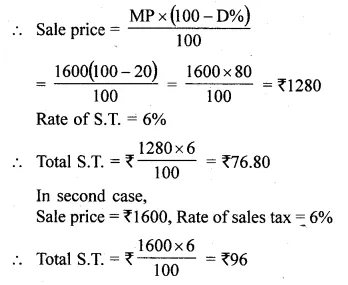(i) Price of camera = ₹ 1600 + ₹ 96 = ₹ 1696
(ii) VAT paid by the shopkeeper= ₹ 96 – ₹ 76.80 = ₹ 19.20

Question 5.
The printed price of an article is ₹ 60000. The wholesaler allows a discount of 20% to the shopkeeper. The shopkeeper sells the article to the customer at the printed price. Sales tax (under VAT) is charged at the rate of 6% at every stage. Find :
(i) the cost to the shopkeeper inclusive of tax.
(ii) VAT paid by the shopkeeper to the Government.
(iii) the cost to the customer inclusive of tax.
Solution:
Printed price of an article = ₹ 60000
Rate of discount allowed = 20%
Total discount = ₹ 60000 x $$\frac { 20 }{ 100 }$$ = ₹ 12000
S.P. after discount = ₹ 60000 – ₹ 12000 = ₹ 48000
Rate of VAT = 6%
(i) Amount paid by the shopkeeper
= ₹ 48000 + ₹ 48000 x $$\frac { 6 }{ 100 }$$
= ₹ 48000 + ₹ 2880 = ₹ 50880
(ii) The price at which the shopkeeper sold to the customer = ₹ 60000
Profit = ₹ 60000 – ₹ 48000 = ₹ 12000
VAT paid by the customer to the Govt.
= ₹ 12000 x $$\frac { 6 }{ 100 }$$ = ₹ 720
(iii) Total cost to the customer = ₹ 60000 + VAT inclusive of tax
= ₹ 60000 + $$\frac { 60000 x 6 }{ 100 }$$
= ₹ 60000 + ₹ 3600 = ₹ 63600

Question 6.
A shopkeeper bought a TV at a discount of 30% of the listed price of ₹ 24000. The shopkeeper offers a discount of 10% of the listed price to his customer. If the VAT (Value Added Tax) is 10%, find : the amount paid by the customer, the VAT to be paid by the shopkeeper.
Solution:
List price = ₹ 24000
Discount = 30%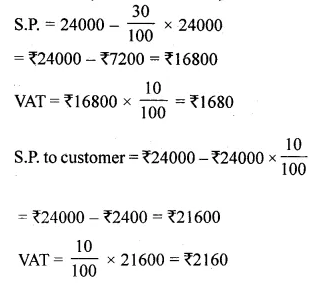(i) Amount paid by customer = ₹ 21600 + ₹ 2160 = ₹ 23760
(ii) Total VAT to be paid by shopkeeper = ₹ 2160 – ₹ 1680 = ₹ 480

Question 7.
A shopkeeper sells an article at the listed price of ₹ 1500 and the rate of VAT is 12% at each stage of sale. If the shopkeeper pays a VAT of ₹ 36 to the Government, what was the amount inclusive of tax at which the shopkeeper purchased the articles from the wholesaler?
Solution:
List price (M.P.) of an article = ₹ 1500
Rate of VAT = 12%
Total VAT = ₹ $$\frac { 1500 x 12 }{ 100 }$$ = ₹ 180
But VAT paid by the shopkeeper = ₹ 36
Total VAT paid by wholeseller = ₹ 180 – ₹ 36 = ₹ 144
Rate of VAT = 12%
S.P. of the whole seller = $$\frac { 144 x 100 }{ 12 }$$ = ₹ 1200
Total amount paid by the wholeseller including VAT = ₹ 1200 + ₹ 144 = ₹ 1344

Question 8.
A shopkeeper buys an article whose list price is ₹ 800 at some rate of discount from a wholesaler. He sells the article to a consumer at the list price and charges sales tax at the prescribed rate of 7.5%. If the shopkeeper has to pay a VAT of ₹ 6, find the rate of discount at which he bought the article from the wholesaler.
Solution:
List price (MP) of an article = ₹ 800
S.P. of the shopkeeper = ₹ 800
Rate of VAT = 7.5%
Total VAT = ₹ $$\frac { 800 x 7.5 }{ 100 }$$ = ₹ 60
VAT paid by the shopkeeper = ₹ 6
VAT paid by the wholeseller = ₹ 60 – ₹ 6 = ₹ 54
Rate of VAT = 7.5%
S.P. of wholesellerQuestion 9.
A manufacturing company ‘P’ sells a Desert cooler to a dealer A for ₹ 8100 including sales tax (under VAT). The dealer A sells it to a dealer B for ₹ 8500 plus sales tax and the dealer B sells it to a consumer at a profit of ₹ 600. If the rate of sales tax (under VAT) is 8%, find
(i) the cost price of the cooler for the dealer A.
(ii) the amount of tax received by the Government.
(iii) the amount which the consumer pays for the cooler.
Solution:
Manufactures ‘P’ selling price for Desert cooler including sales tax (VAT) = ₹ 8100
Rate of sales tax (VAT) = 8%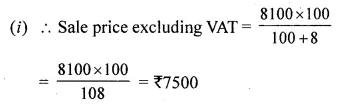Cost price of dealer A = ₹ 7500
and sale price of dealer A = ₹ 8500
Gain = ₹ 8500 – ₹ 7500 = ₹ 1000
or cost price of dealer B = ₹ 8500
Gain = ₹ 600
S.P. of dealer B = ₹ 8500 + ₹ 600 = ₹ 9100
Consumers cost price = ₹ 8500 + ₹ 600 = ₹ 9100
(ii) Tax paid to the Govt.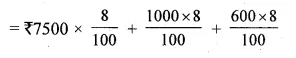= ₹ 600 + ₹ 80 + ₹ 48 = ₹ 728
The amount which the consumer pays = ₹ 7500 + ₹ 1000 + ₹ 600 + ₹ 728 = ₹ 9828

Question 10.
A manufacturer marks an article for ₹ 5000. He sells it to a wholesaler at a discount of 25% on the marked price and the wholseller sells it to a retailer at a discount of 15% on the marked price. The retailer sells it to a consumer at the marked price and at each stage the VAT is 8%.
Calculate the amount of VAT received by the Government from :
(i) the wholesaler.
(ii) the retailer.
Solution:
Marked price (M.P.) of an article = ₹ 5000
Discount given to the wholesaler = 25%
Cost price of wholesaler or S.P. of the manufacturer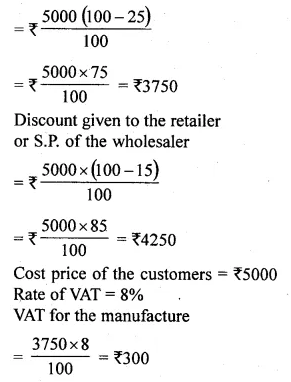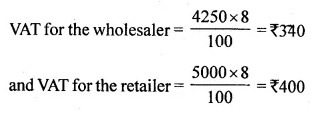(i) VAT received from the wholesaler = ₹ 340 – ₹ 300 = ₹ 40
(ii) and VAT received by the retailer = ₹ 400 – ₹ 340 = ₹ 60

Question 11.
A manufacturer listed the price of his goods at ₹ 160 per article. He allowed a discount of 25% to a wholesaler who in his turn allowed a discount of 20% on the listed price to a retailer. The rate of sales tax on the goods is 10%. If the retailer sells one article to a consumer at a discount of 5% on the listed price, then find
(i) the VAT paid by the wholesaler.
(ii) the VAT paid by the retailer.
(iii) the VAT received by the Government.
Solution:
List price (MP) of the goods = ₹ 160 per article
Rate of discount = 25%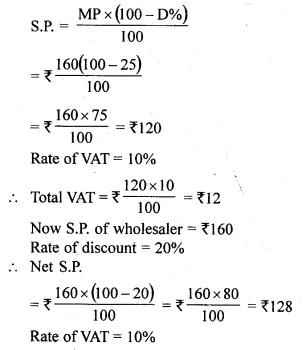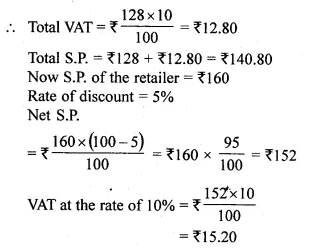(i) VAT paid by the wholesaler = ₹ 12.80 – ₹ 12 = ₹ 0.80
(ii) VAT paid by the retailer = 15.20 – 12.80 = ₹ 2.40
(iii) Total VAT paid to the Govt. = ₹ 15.20

Question 12.
Kiran purchases an article for ₹ 5, 400 which includes 10% rebate on the marked price and 20% sales tax (under VAT) on the remaining price. Find the marked price of the article.
Solution:
Let market price of the article be ₹ x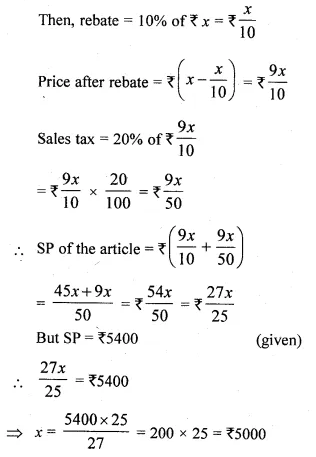Hence, market price of the article is ₹ 5000

Question 13.
A shopkeeper buys an article for ₹ 12000 and marks up its price by 25%. The shopkeeper gives a discount of 10% on the marked up price. He gives a further off-season discount of 5% on the balance. But the sales tax (under VAT) is charged at 8% on the remaining price. Find :
(i) the amount of VAT which a customer has to pay.
(ii) the final price he has to pay for the article.
Solution:
Cost price of an article = ₹ 12000
Rate of mark up in price = 25%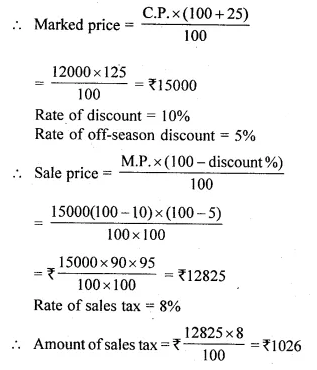(i) Amount of sales tax = ₹ 1026
(ii) Price to be paid = ₹ 12825 + ₹ 1026 = ₹ 13851

Question 14.
In a particular tax period, Mr. Sunder Dass, a shopkeeper pruchased goods worth ₹ 960000 and paid a total tax of ₹ 62750 (under VAT). During this period, his sales consisted of taxable turnover of ₹ 400000 of goods taxable at 6% and ₹ 480000 for goods taxable at 12.5%. He also sold tax exempted goods worth ₹ 95640 in the same period. Calculate his tax liability (under VAT) for this period.
Solution:
Cost price of good purchased by Sunder Dass = ₹ 960000
Tax paid (VAT) = ₹ 62750
Sale of goods worth = ₹ 400000
Rate of VAT = 6%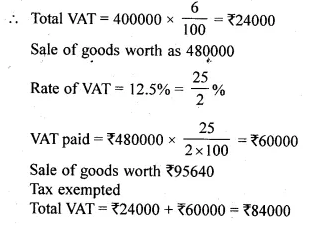Tax paid to Govt. (VAT) = ₹ 62750
Tax liability = ₹ 84000 – ₹ 62750 = ₹ 21250

Question 15.
In the tax period ended March 2015, M/S Hari Singh & Sons purchased floor tiles worth ₹ 800000 taxable at 7.5% and sanitary fittings worth ₹ 750000 taxable at 10%. During this period, the sales turnover for floor tiles and sanitary fittings are worth ₹ 840000 and ₹ 920000 respectively. However, the floor tiles worth ₹ 60000 were returned by the firm during the same period. Calculate the tax liability (under VAT) of the firm for this tax period.
Solution:
Cost of floor tiles = ₹ 800000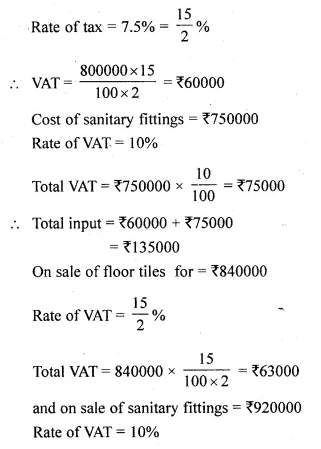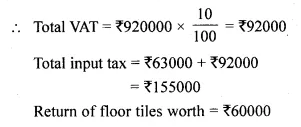Liability of tax of the firm = 155000 – (135000 + 4500) = ₹ 155000 – ₹ 139500 = ₹ 15500

Hope given ML Aggarwal Class 10 Solutions for ICSE Maths Chapter 1 Value Added Tax EX 1 are helpful to complete your math homework.

If you have any doubts, please comment below. Learn Insta try to provide online math tutoring for you.